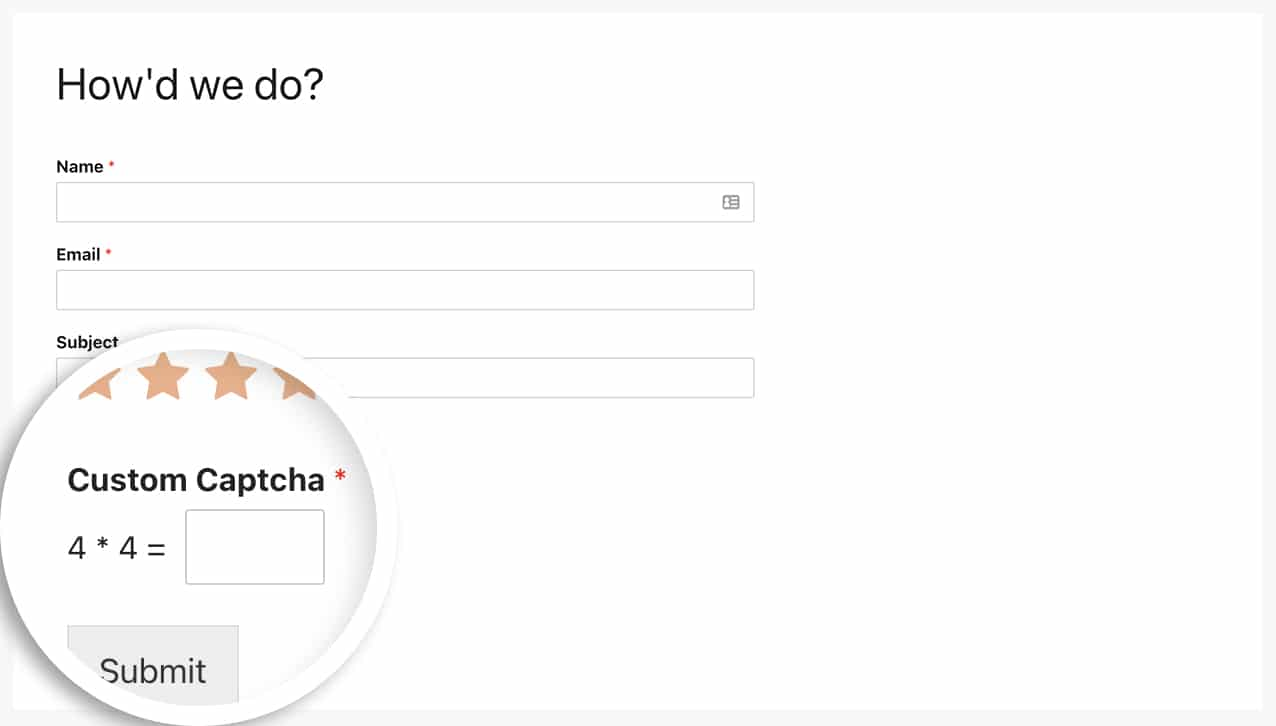# How to Change the Captcha Math for the Custom Captcha Field

## Introduction

Would you like to change the captcha math for your visitors with the Custom Captcha field? If you wish to change the math used or limit the range of numbers used in the equation or to limit the calculation method itself, you can easily do this with a custom code snippet.

By default, the Custom Captcha field uses addition and multiplication for it’s math with number ranging from 1 to 15.

In this tutorial, we’ll show you how to use PHP to change the minimum and maximum numbers used as well as the calculation method using PHP.

## Creating the form

To begin, you’ll need to create a new form and add your Custom Captcha form field to the form.

If you need any help in how to set up a form using the Custom Captcha form field, please review this documentation.Below, we’re going to show you two different snippets. One will just change the maximum number that the equation uses and one will change the calculation to additional only.

Simply choose which one of the options below you’d like to use and add it to your site.

If you need any assistance in how to add snippets to your site, please review this tutorial.

#### Changing the min number

The example shown below will set the minumum number for the equation to 2.

```/**
*
*/

/* Default settings
array(
'min' => 1,
'max' => 15,
'cal' => array( '+', '*', '-' ),
);
*/

// Change min number 2 for easier math
\$settings[ 'min' ] = 2;

return \$settings;
}

```

#### Changing the max number

The example shown below will set the maximum number for the equation to 4.

```/**
*
*/

/* Default settings
array(
'min' => 1,
'max' => 15,
'cal' => array( '+', '*', '-' ),
);
*/

// Change max number 4 for easier math
\$settings[ 'max' ] = 4;

return \$settings;
}

```

#### Using only multiplication

In this example, we’re changing the calculation to only use multiplication.

```/**
*
*/

/* Default settings
array(
'min' => 1,
'max' => 15,
'cal' => [ '+', '*', '-' ],
);
*/

// Use multiplication only for calculations
\$settings[ 'cal' ] = [ '*' ];

return \$settings;
}
```And that’s it! With this code snippet, you can now change the numbers or the calculation used in the Custom Captcha field. Would you like to limit the number of characters a user can enter in your form field? Have a look at our article on How to Limit the Number of Characters for a Text Field.

## FAQ

#### Q: How can I change all of them in the same function?

A: You can absolutely include the minimum, maximum and calculation all in one function by using this snippet.

```function wpf_dev_math_captcha_settings( \$settings ) {

/* Default settings
array(
'min' => 1,
'max' => 15,
'cal' => [ '+', '*' ],
);
*/

// Use addition only for easier math
// Use the minimum number of 1
// Use the maximum number of 10
\$settings[ 'cal' ] = [ '+' ];
\$settings[ 'min' ] = [ '1' ];
\$settings[ 'max' ] = [ '10' ];

return \$settings;
}